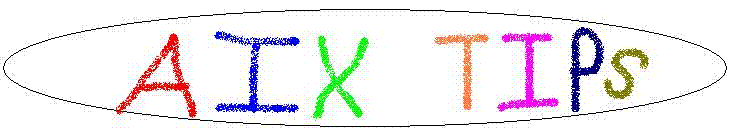### AIX Tip of the Week: ANSI Terminal Support

Date: April 5, 2000

The default AIX installation does not include support for ANSI terminals. ANSI support is important because Win95/98 telnet clients use this terminal emulation. Without ANSI support, full screen applications such as smit or vi, won't work properly. To support ANSI terminals, install bos.terminfo.ansi.data package on the base AIX CD.

As an alternative, the ANSI terminfo file is attached below. To install:

1. cp ansi.ti /usr/lib/terminfo
2. tic /usr/lib/terminfo/ansi.ti

## ansi.ti

```# @(#)18    1.1  src/bos/usr/share/lib/terminfo/ansi.ti, terminfo, bos430, 9737A_430 9/1/93 20:34:12
#
# COMPONENT_NAME: (TERMINFO) Terminfo
#
# FUNCTIONS:    ansi.ti
#
#   ORIGINS: 4
#
#   OBJECT CODE ONLY SOURCE MATERIALS
#
# ident	"@(#)terminfo:ansi.ti	1.12"
# ANSI - standards and other nonexistent terminals emulated in software.
#
# Manufacturer:	ANSI - Generic
# Class:	III
#
# Info:
#	This category is used for terminals people "make up" in software
#	that do not represent a particular piece of hardware. This
#	includes standards, such as the ANSI standard, as well as
#	emulator programs that accept a particular set of escape
#	sequences.
#
#	ANSI capabilities are broken up into pieces, so that a terminal
#	implementing some ANSI subset can use many of them.
#
ansi+local,
cud=\E[%p1%dB, cub=\E[%p1%dD, cuf=\E[%p1%dC, cuu=\E[%p1%dA,
ansi+local1,
cud1=^J, cub1=^H, cuf1=\E[C, cuu1=\E[A,
ansi+tabs,
ht=^I, cbt=\E[Z, hts=\EH, tbc=\E[2g,
ansi+inittabs,
it#8, use=ansi+tabs,
ansi+erase,
ed=\E[J, el=\E[K, clear=\E[H\E[J,
ansi+rca,
hpa=\E[%p1%{1}%+%dG, vpa=\E[%p1%{1}%+%dd,
ansi+cup,
cup=\E[%i%p1%d;%p2%dH, home=\E[H,
ansi+rep,
rep=%p1%c\E[%p2%{1}%-%db,
ansi+idl,
dl=\E[%p1%dM, il=\E[%p1%dL,
ansi+idl1,
dl1=\E[M, il1=\E[L,
ansi+idc,
dch1=\E[P, ich=\E[%p1%d@, ich1=\E[@,
#	smir=\E6, rmir=\E6,	commented out by ehr3
ansi+arrows,
kcuu1=\E[A, kcud1=\E[B, kcub1=\E[D, kcuf1=\E[C, khome=\E[H, kbs=^H,
ansi+sgr|ansi graphic renditions,
ansi+sgrso|ansi standout only,
rmso=\E[m, smso=\E[7m,
ansi+sgrul|ansi underline only,
rmul=\E[m, smul=\E[4m,
ansi+sgrbold|ansi graphic renditions; assuming terminal has bold; not dim,
bold=\E[1m,
sgr=\E[%?%p1%t7;%;%?%p2%t4;%;%?%p3%t7;%;%?%p4%t5;%;%?%p6%t1;%;m,
use=ansi+sgr, use=ansi+sgrso, use=ansi+sgrul,
ansi+sgrdim|ansi graphic renditions; assuming terminal has dim; not bold,
dim=\E[2m,
sgr=\E[%?%p1%t7;%;%?%p2%t4;%;%?%p3%t7;%;%?%p4%t5;%;%?%p5%t2;%;m,
use=ansi+sgr, use=ansi+sgrso, use=ansi+sgrul,
ansi+pp|ansi printer port,
mc4=\E[4i, mc5=\E[5i, mc0=\E[0i,
# Info:
#	ANSI is a vanilla ANSI terminal. This is assumed to implement
#	all the normal ANSI stuff with no extensions. It assumes
#	insert/delete line/char is there, so it won't work with
#	vt100 clones. It assumes video attributes for bold, blink,
#	underline, and reverse, which won't matter much if the terminal
#	can't do some of those. Padding is assumed to be zero, which
#	shouldn't hurt since xon/xoff is assumed.
#
#	We assume a 24x80 screen. This entry was derived from the
#	Ann Arbor Ambassador, and is untested.
#
ansi|generic ansi standard terminal,
use=vanilla, am, cols#80, lines#24, xon,
use=ansi+cup, use=ansi+rca,
use=ansi+erase,
use=ansi+tabs,
use=ansi+local1, use=ansi+local,
use=ansi+idc, use=ansi+idl1, use=ansi+idl,
use=ansi+rep,
use=ansi+sgrbold, use=ansi+arrows,
# Info:
#	Bare minimum ANSI terminal. This should work on anything, but
#	beware of screen size problems and memory relative cursor
#
minansi|minimum ansi standard terminal,
am, xon, use=vanilla, cols#80, lines#24,
use=ansi+cup, use=ansi+erase,
# Info:
#	This terminal type is for ANSI terminals with ONLY memory
#	relative cursor addressing and more than one page of memory.
#	and makes almost no assumptions. It does assume auto margins,
#	no padding and/or xon/xoff, and a 24x80 screen.
#
mransi|mem rel cup ansi,
am, use=vanilla, cols#80, lines#24, xon,
use=ansi+erase, use=ansi+local1,
# Info:
#	Columbus UNIX virtual terminal. This terminal also appears in
#	UNIX 4.0 and successors as line discipline 1 (?), but is
#	undocumented and does not really work quite right.
#
virtual|cbunix|cb-unix|cb-unix virtual terminal,
cr=^M, cud1=^J, ind=^J, bel=^G, cols#80, lines#24, am, clear=\EJ,
cub1=^H, cup=\EG%p2%c%p1%c, cuf1=\EC, cuu1=\EA, el=\EK,
ed=\EL, il1=\EP, dl1=\EN, ich1=\EO, dch1=\EM, lm#0, da, db,
kcub1=\ED, kcuf1=\EC, kcuu1=\EA, kcud1=\EB, khome=\EE,
smso=\Ea\004, rmso=\Eb\004, smul=\Ea\001, rmul=\Eb\001,
# Info:
#	This terminal is based on virtual but cleans up a few problems
#	with control characters in parameter strings. It is implemented
#	in mrh's window manager.
#
pty|4bsd pty terminal,
smso=\Ea\$, rmso=\Eb\$, smul=\Ea!, rmul=\Eb!,
cup=\EG%p1%' '%+%c%p2%' '%+%c, use=virtual,
# Info:
#	A dumb terminal with 1 line which is a fake status line.
#	This is useful to run sysline in in a multi-window environment.
#
1line|one_line|one line window,
cr=^M, ind=^J, cols#80, lines#1, am, ht=^I, cub1=^H,
hs, tsl=\n,
# Info:
#	1linepty is like 1line but the 1 line window is smarter,
#	with standout, cursor addressing, and clear to eol.
#
1linepty|one_linepty|1 line window in a pty,
smso=\Ea\$, rmso=\Eb\$, smul=\Ea!, rmul=\Eb!,
cup=\EG%p1%' '%+%c%p2%' '%+%c, tsl=\r\EK\EG %p2%' '%+%c,
eslok, use=1line,
vanilla,
bel=^G, cr=^M, cud1=^J, ind=^J,
datakit,
am, gn,
cols#80,
bel=^G, cr=\r, cud1=\n, ind=\n,

```